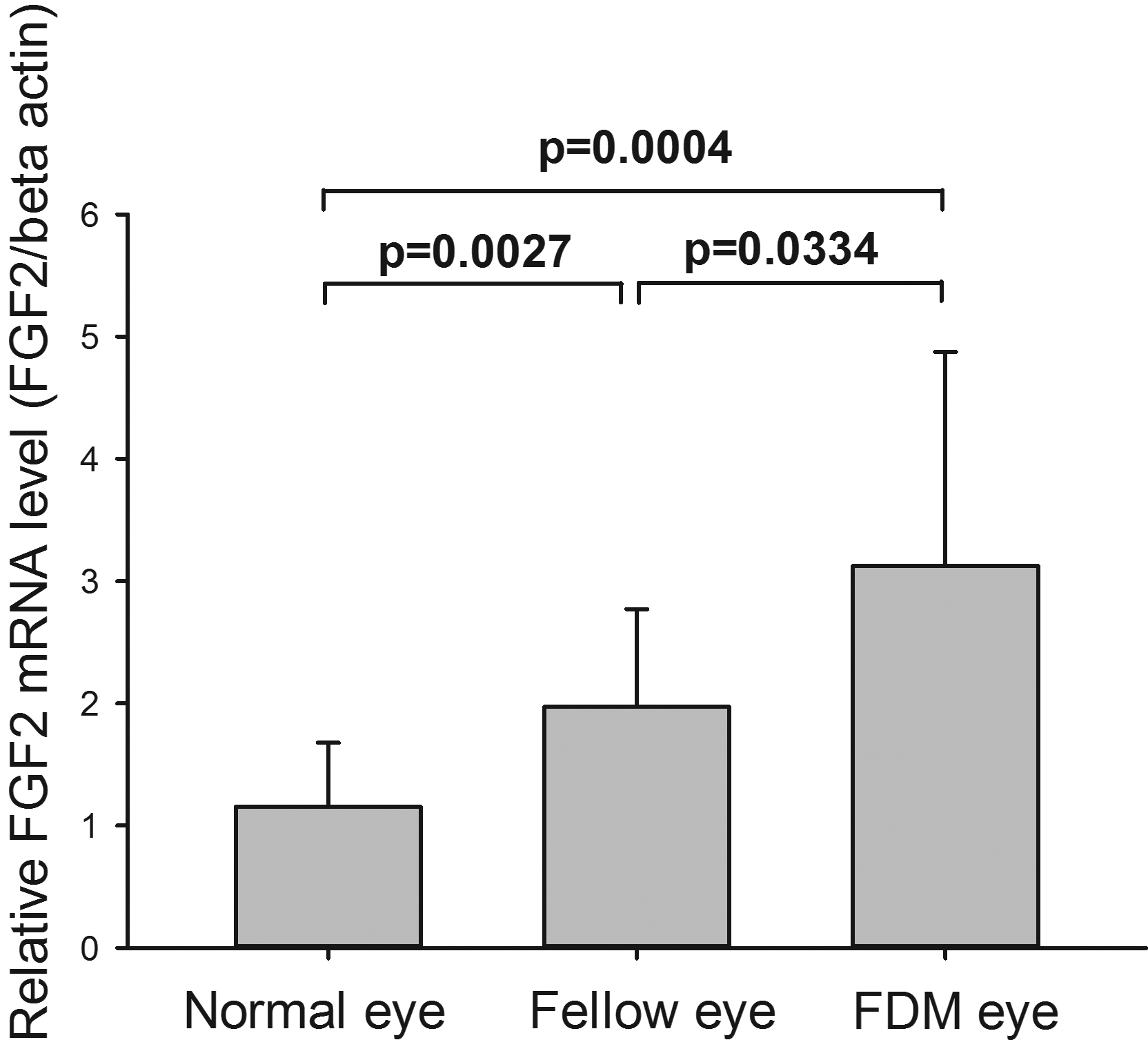Figure 1 of An, Mol Vis 2012; 18:471-478.

Figure 1. Relative FGF2 mRNA level in FDM, fellow and normal eyes. The expression levels of FDM eye (n=14) and fellow eye (n=14) were significantly higher than normal eye (n=13, the averaged data from both eyes was used for each animal; p=0.00004 and 0.0027, respectively). The expression level of FDM eye was also higher than the fellow eye (p=0.0334). The expression level of normal eye was the expression level of right (n=13) eyes of normal control guinea pigs. The test of significance in comparing FDM and fellow eyes was using paired-t statistics, and unpaired t-test for the comparison between eyes of normal controls and eyes of form deprivation group.